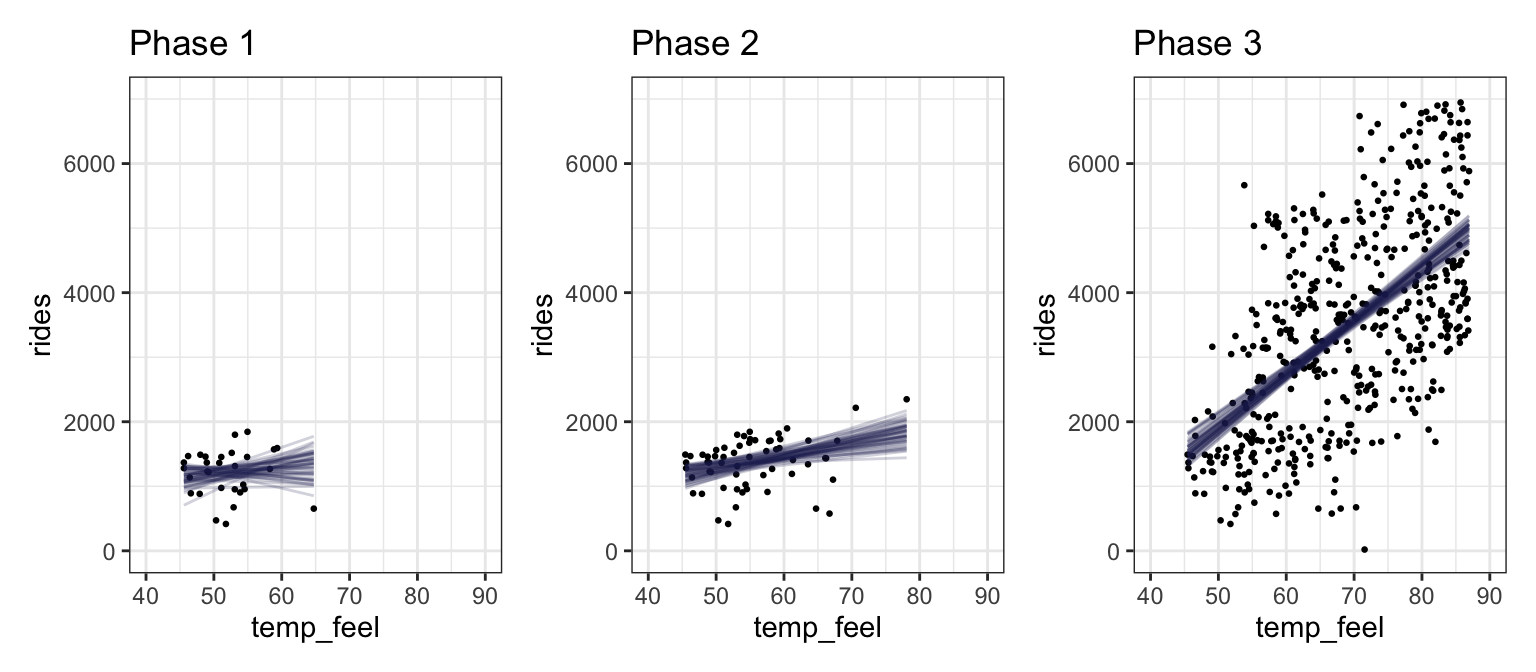Published

October 4, 2022

(Original chapter)

library(bayesrules)
library(tidyverse)
library(brms)
library(cmdstanr)
library(rstanarm)
library(broom.mixed)
library(tidybayes)
library(ggdist)
library(patchwork)
library(ggtext)

# Plot stuff
clrs <- MetBrewer::met.brewer("Lakota", 6)
theme_set(theme_bw())

# Seed stuff
set.seed(1234)
BAYES_SEED <- 1234

data(bikes, package = "bayesrules")

bikes <- bikes |>
mutate(temp_feel_centered = scale(temp_feel, scale = FALSE),
temp_feel_c = as.numeric(temp_feel_centered))

temp_details <- attributes(bikestemp_feel_centered) %>% set_names(janitor::make_clean_names(names(.))) ## 9.2: Tuning prior models for regression parameters We want to estimate the effect of temperature on bike share ridership. We need to estimate three parameters: 1. $$\beta_0$$, or the intercept. From prior research, we know: On an average temperature day, say 65 or 70 degrees for D.C., there are typically around 5000 riders, though this average could be somewhere between 3000 and 7000. 1. $$\beta_1$$, or the slope. From prior research, we know: For every one degree increase in temperature, ridership typically increases by 100 rides, though this average increase could be as low as 20 or as high as 180. 1. $$\sigma$$, or the variation in ridership. From prior research, we know: At any given temperature, daily ridership will tend to vary with a moderate standard deviation of 1250 rides. The intercept there is centered at 5000 riders. That gives us these priors: p1 <- ggplot() + geom_function(fun = ~dnorm(., 5000, 1000), size = 1, color = clrs) + xlim(c(1000, 9000)) + labs(x = "**β<sub>0c</sub>**<br>Average daily ridership, centered") + theme(axis.title.x = element_markdown()) ## Warning: Using size aesthetic for lines was deprecated in ggplot2 3.4.0. ## ℹ Please use linewidth instead. p2 <- ggplot() + geom_function(fun = ~dnorm(., 100, 40), size = 1, color = clrs) + xlim(c(-50, 250)) + labs(x = "**β<sub>1</sub>**<br>Effect of temperature on ridership") + theme(axis.title.x = element_markdown()) p3 <- ggplot() + geom_function(fun = ~dexp(., 1 / 1250), size = 1, color = clrs) + xlim(c(0, 7000)) + labs(x = "**σ**<br>Variation in daily ridership") + theme(axis.title.x = element_markdown()) p1 | p2 | p3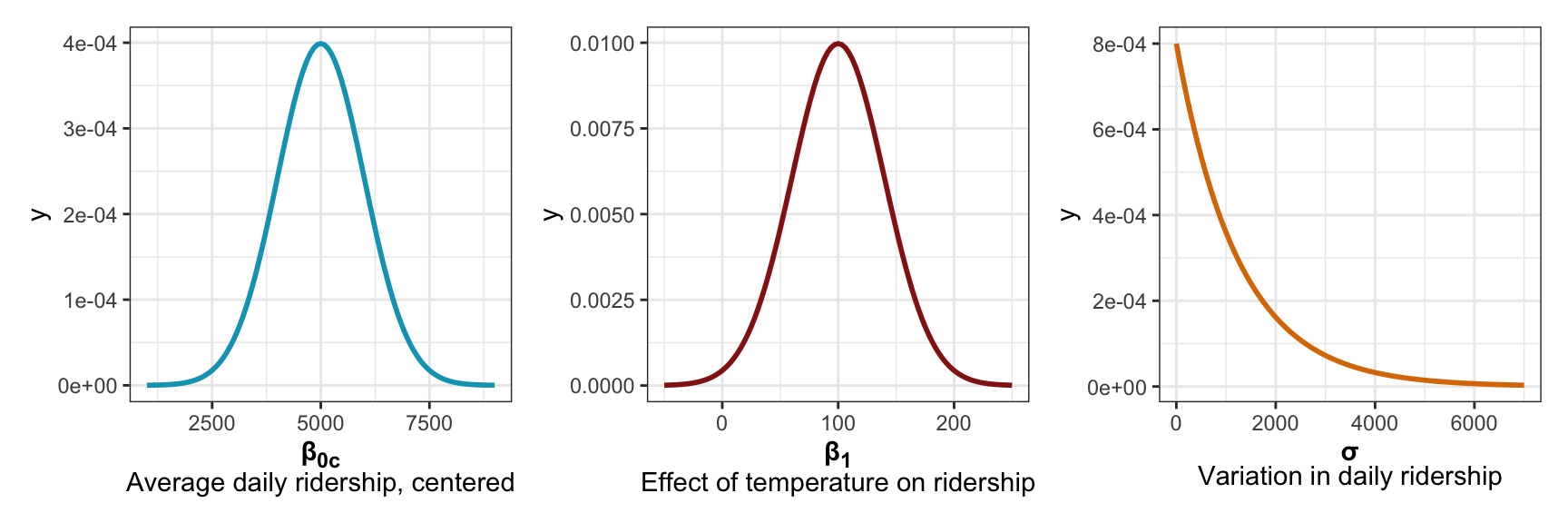More formally, we can write out the whole model like this: \begin{aligned} Y_i &\sim \mathcal{N}(\mu_i, \sigma) \text{, or} & \text{[McElreath's syntax]} \\ Y_i \mid \beta_0, \beta_1, \sigma &\stackrel{\text{ind}}{\sim} \mathcal{N}(\mu_i, \sigma^2) & \text{[Bayes Rules!'s syntax]} \\ \mu_i &= \beta_{0c} + \beta_1 X_i \\ \\ \beta_{0c} &\sim \mathcal{N}(5000, 1000) \\ \beta_{1} &\sim \mathcal{N}(100, 40) \\ \sigma &\sim \operatorname{Exponential}(1 / 1250) \end{aligned} We can simulate from all these priors to see how reasonable they are, McElreath-style. In Bayes Rules! they show a picture of this but not the code to make it. I’ll use brms bc it’s easy. priors <- c(prior(normal(5000, 1000), class = Intercept), prior(normal(100, 40), class = b, coef = "temp_feel_c"), prior(exponential(0.0008), class = sigma)) bike_prior_only <- brm( bf(rides ~ temp_feel_c), data = bikes, family = gaussian(), prior = priors, sample_prior = "only", backend = "cmdstanr", cores = 4, seed = BAYES_SEED, refresh = 0 ) ## Start sampling These lines all look reasonable, yay. draws_prior <- tibble(temp_feel_c = seq(45 - temp_detailsscaled_center,
90 - temp_details$scaled_center, 1)) |> add_epred_draws(bike_prior_only, ndraws = 200) |> mutate(unscaled = temp_feel_c + temp_details$scaled_center)

draws_prior |>
ggplot(aes(x = unscaled, y = .epred)) +
geom_line(aes(group = .draw), alpha = 0.2) +
labs(x = "Temperature", y = "Number of rides")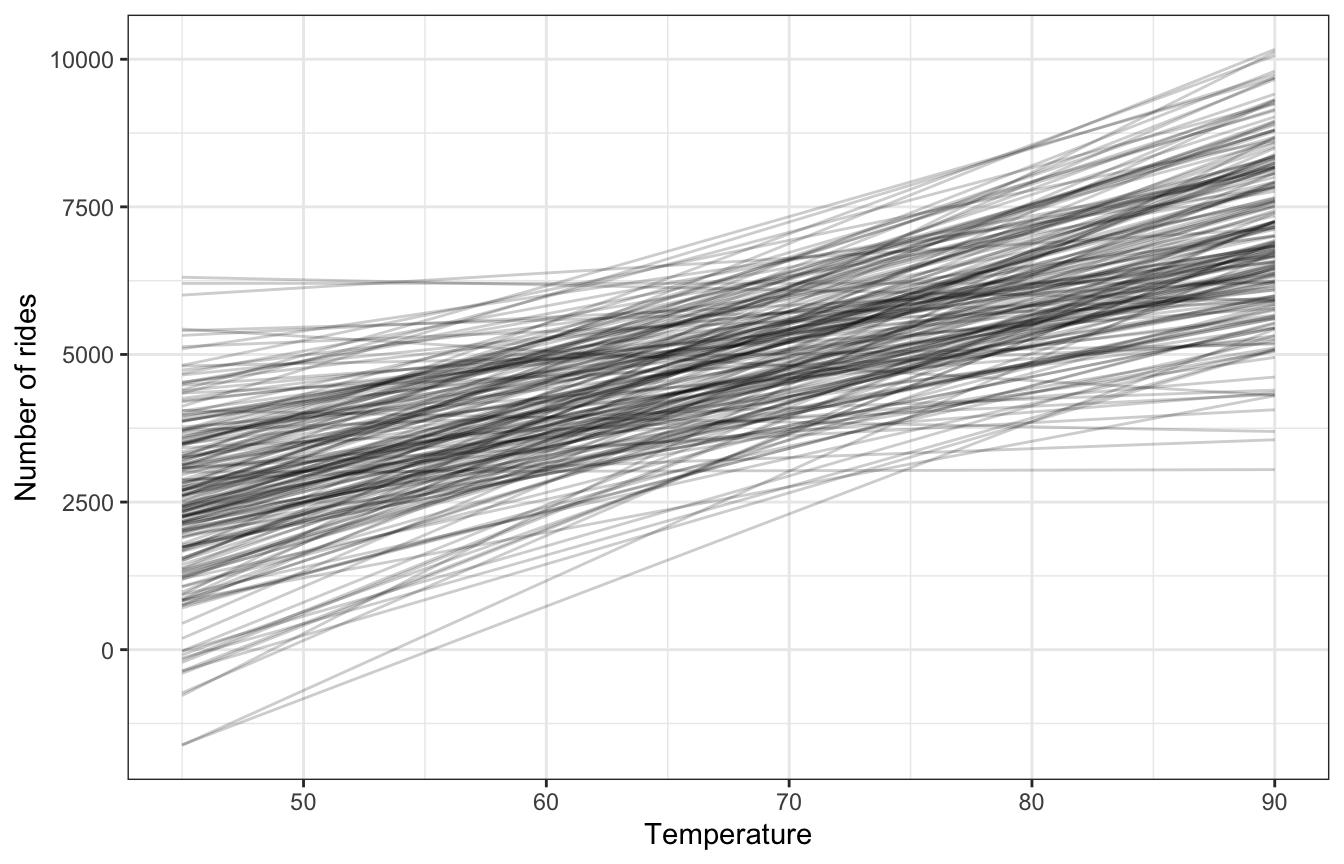## 9.3: Posterior simulation

### Run the model

bike_rstanarm <- stan_glm(
rides ~ temp_feel_c,
data = bikes,
family = gaussian(),
prior_intercept = normal(5000, 1000),
prior = normal(100, 40),
prior_aux = exponential(0.0008),
chains = 4, iter = 5000*2, seed = 84735, refresh = 0
)
priors <- c(prior(normal(5000, 1000), class = Intercept),
prior(normal(100, 40), class = b, coef = "temp_feel_c"),
prior(exponential(0.0008), class = sigma))

bike_brms <- brm(
bf(rides ~ temp_feel_c),
data = bikes,
family = gaussian(),
prior = priors,
chains = 4, iter = 5000*2, seed = BAYES_SEED,
backend = "cmdstanr", refresh = 0,
file = "09-manual_cache/bike-brms"
)
## Start sampling
09-stan/bike-simple.stan
data {
int<lower = 0> n;
vector[n] Y;
vector[n] X;
}

/*
// We could also center things here and then use it in mu below:
// mu = beta0 + beta1 * X_centered;
// See https://mc-stan.org/docs/stan-users-guide/standardizing-predictors-and-outputs.html
transformed data {
vector[n] X_centered;

X_centered = X - mean(X);
}
*/

parameters {
real beta0;
real beta1;
real<lower = 0> sigma;
}

transformed parameters {
vector[n] mu;
mu = beta0 + beta1 * X;
}

model {
Y ~ normal(mu, sigma);

beta0 ~ normal(5000, 1000);
beta1 ~ normal(100, 40);
sigma ~ exponential(0.0008);
}

generated quantities {
vector[n] Y_rep;

for (i in 1:n) {
Y_rep[i] = normal_rng(mu[i], sigma);
}
}
bike_stan <- cmdstan_model("09-stan/bike-simple.stan")
bike_stan_samples <- bike_stan$sample( data = list(n = nrow(bikes), Y = bikes$rides, X = bikes$temp_feel_c), parallel_chains = 4, iter_warmup = 5000, iter_sampling = 5000, refresh = 0, seed = BAYES_SEED, output_dir = "09-stan" ) ## Running MCMC with 4 parallel chains... ## ## Chain 1 finished in 2.0 seconds. ## Chain 3 finished in 2.0 seconds. ## Chain 4 finished in 2.0 seconds. ## Chain 2 finished in 2.0 seconds. ## ## All 4 chains finished successfully. ## Mean chain execution time: 2.0 seconds. ## Total execution time: 2.1 seconds. ### Diagnostics ##### Effective sample size and R-hat neff_ratio(bike_rstanarm) ## (Intercept) temp_feel_c sigma ## 0.96345 0.96075 0.96825 rhat(bike_rstanarm) ## (Intercept) temp_feel_c sigma ## 0.9998765 0.9999727 1.0000086 ##### Trace plots bike_rstanarm |> gather_draws((Intercept), temp_feel_c, sigma) |> ungroup() |> mutate(.variable = fct_relevel(factor(.variable), c("(Intercept)", "temp_feel_c", "sigma"))) |> ggplot(aes(x = .iteration, y = .value, color = as.factor(.chain))) + geom_line(size = 0.05) + labs(color = "Chain") + facet_wrap(vars(.variable), scales = "free_y") + theme(legend.position = "bottom")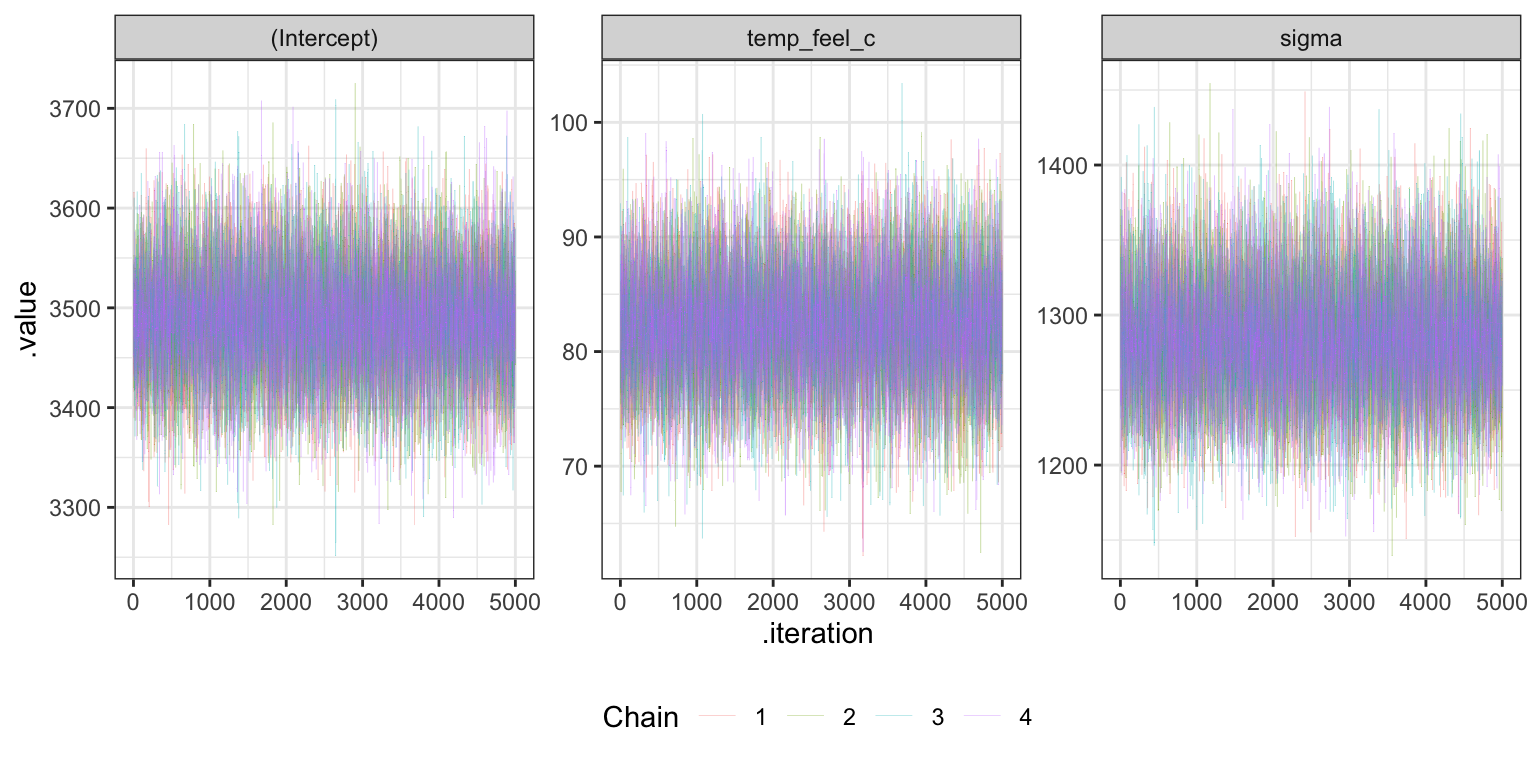##### Trank plots bike_rstanarm |> gather_draws((Intercept), temp_feel_c, sigma) |> ungroup() |> mutate(.variable = fct_relevel(factor(.variable), c("(Intercept)", "temp_feel_c", "sigma"))) |> group_by(.variable) |> mutate(draw_rank = rank(.value)) |> ggplot(aes(x = draw_rank)) + stat_bin(aes(color = factor(.chain)), geom = "step", binwidth = 1000, position = position_identity(), boundary = 0) + labs(color = "Chain") + facet_wrap(vars(.variable)) + theme(legend.position = "bottom", axis.text.y = element_blank(), axis.title.y = element_blank(), axis.ticks.y = element_blank())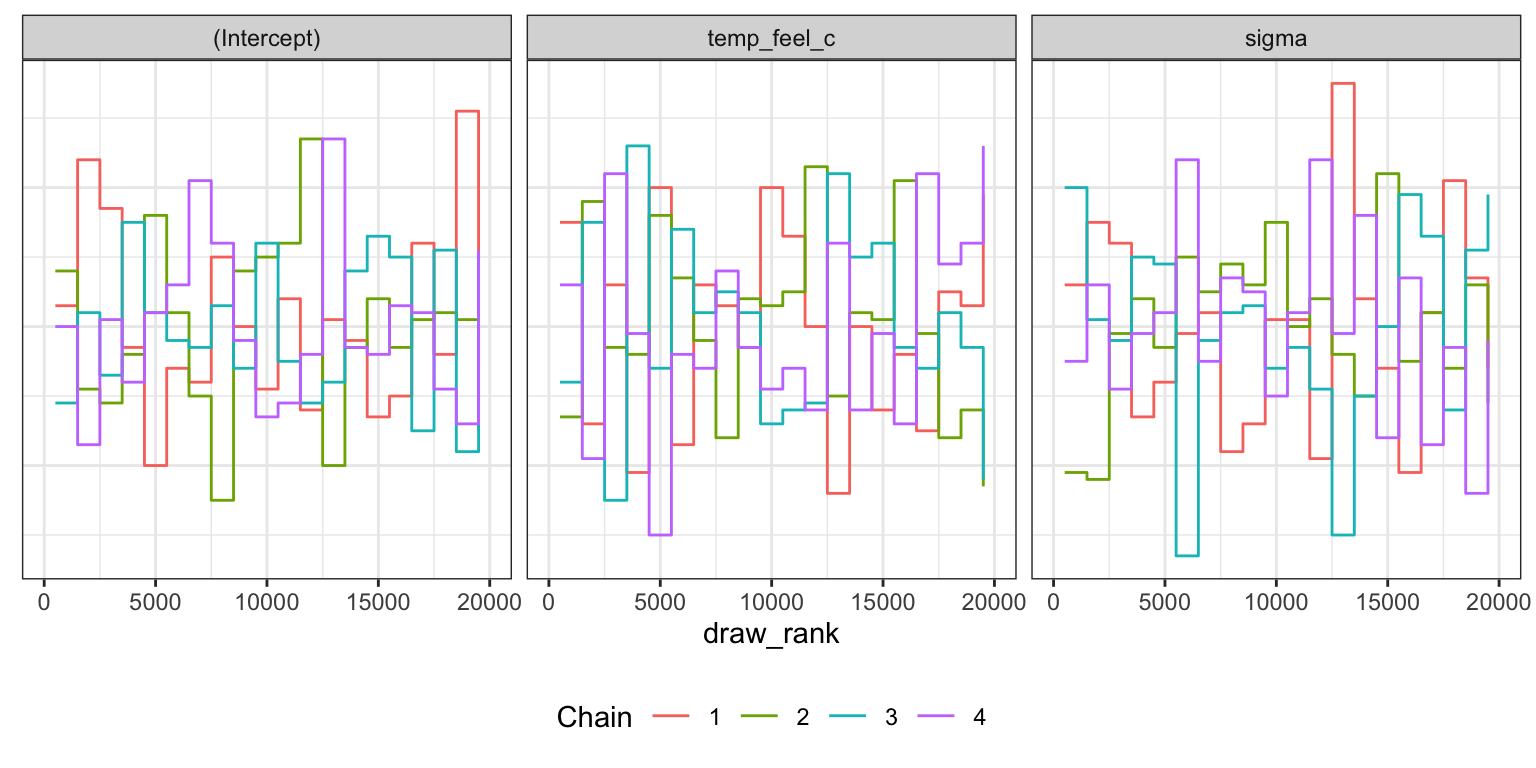##### Density plots bike_rstanarm |> gather_draws((Intercept), temp_feel_c, sigma) |> ungroup() |> mutate(.variable = fct_relevel(factor(.variable), c("(Intercept)", "temp_feel_c", "sigma"))) |> ggplot(aes(x = .value, color = factor(.chain))) + geom_density() + labs(color = "Chain") + facet_wrap(vars(.variable), scales = "free") + theme(legend.position = "bottom")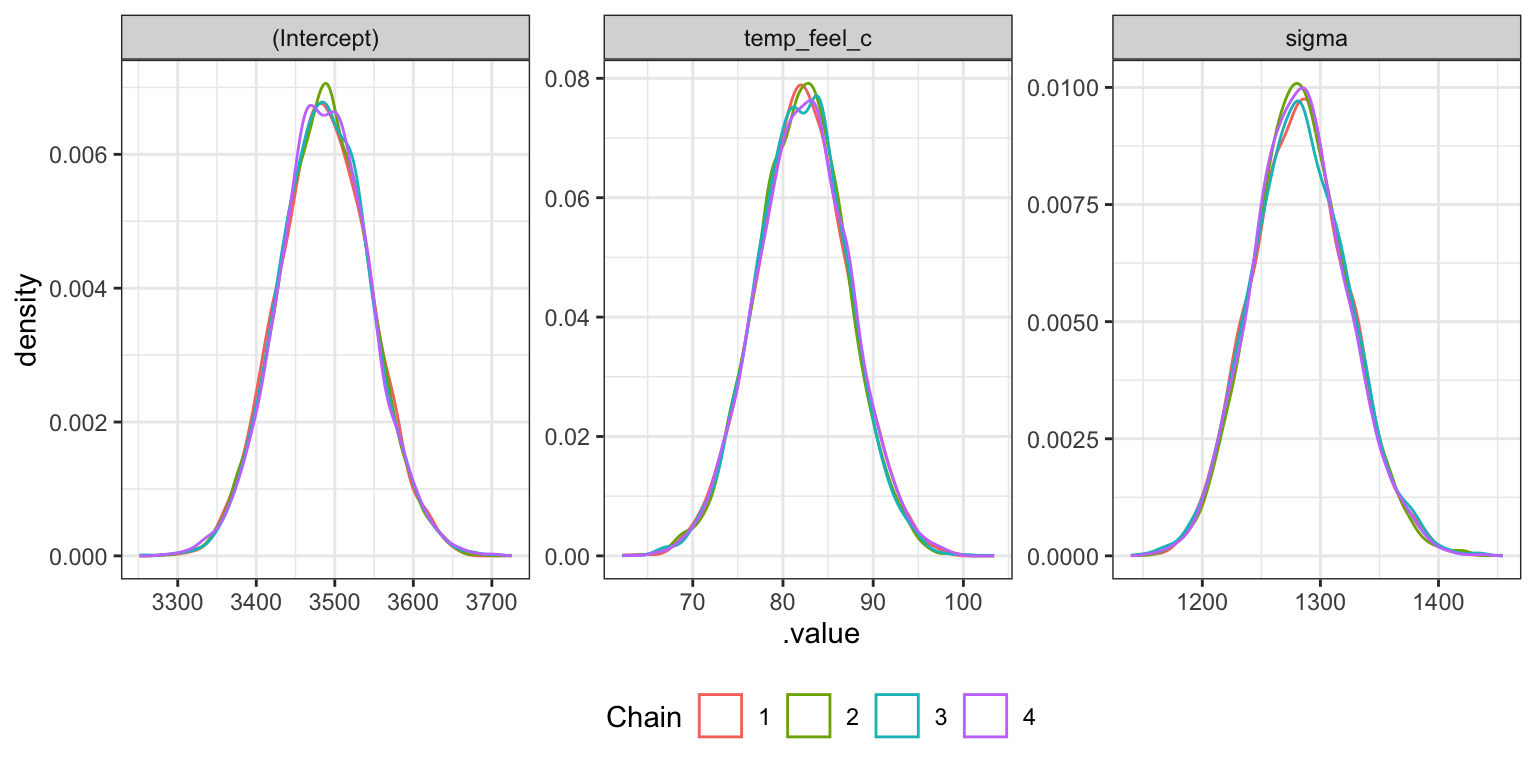##### Effective sample size and R-hat neff_ratio(bike_brms) ## b_Intercept b_temp_feel_c sigma lprior lp__ ## 1.0579051 1.0534749 1.1040274 1.0473025 0.4827253 rhat(bike_brms) ## b_Intercept b_temp_feel_c sigma lprior lp__ ## 1.000021 1.000172 1.000024 1.000135 1.000185 ##### Trace plots bike_brms |> gather_draws(b_Intercept, b_temp_feel_c, sigma) |> ggplot(aes(x = .iteration, y = .value, color = as.factor(.chain))) + geom_line(size = 0.05) + labs(color = "Chain") + facet_wrap(vars(.variable), scales = "free_y") + theme(legend.position = "bottom")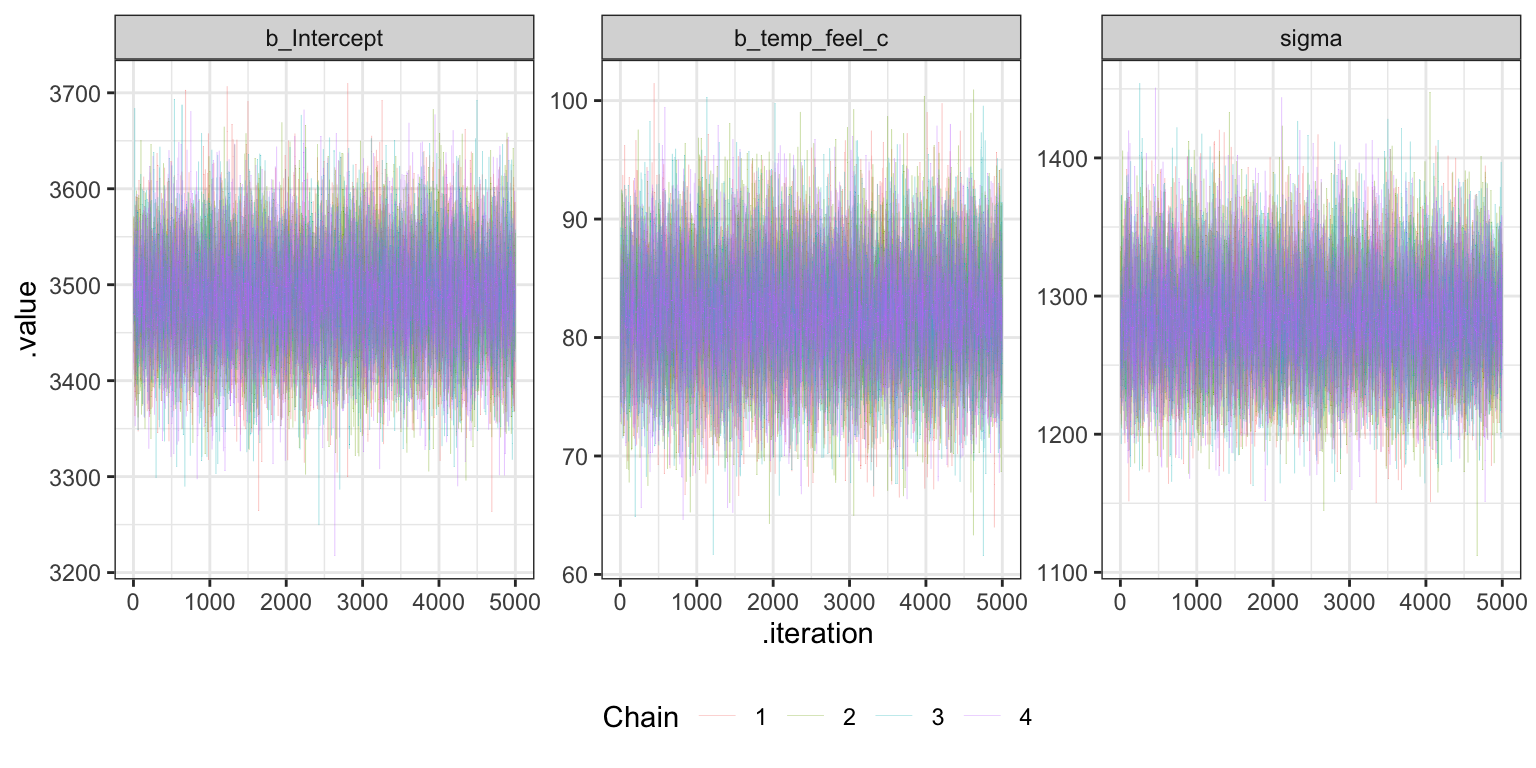##### Trank plots bike_brms |> gather_draws(b_Intercept, b_temp_feel_c, sigma) |> group_by(.variable) |> mutate(draw_rank = rank(.value)) |> ggplot(aes(x = draw_rank)) + stat_bin(aes(color = factor(.chain)), geom = "step", binwidth = 1000, position = position_identity(), boundary = 0) + labs(color = "Chain") + facet_wrap(vars(.variable)) + theme(legend.position = "bottom", axis.text.y = element_blank(), axis.title.y = element_blank(), axis.ticks.y = element_blank())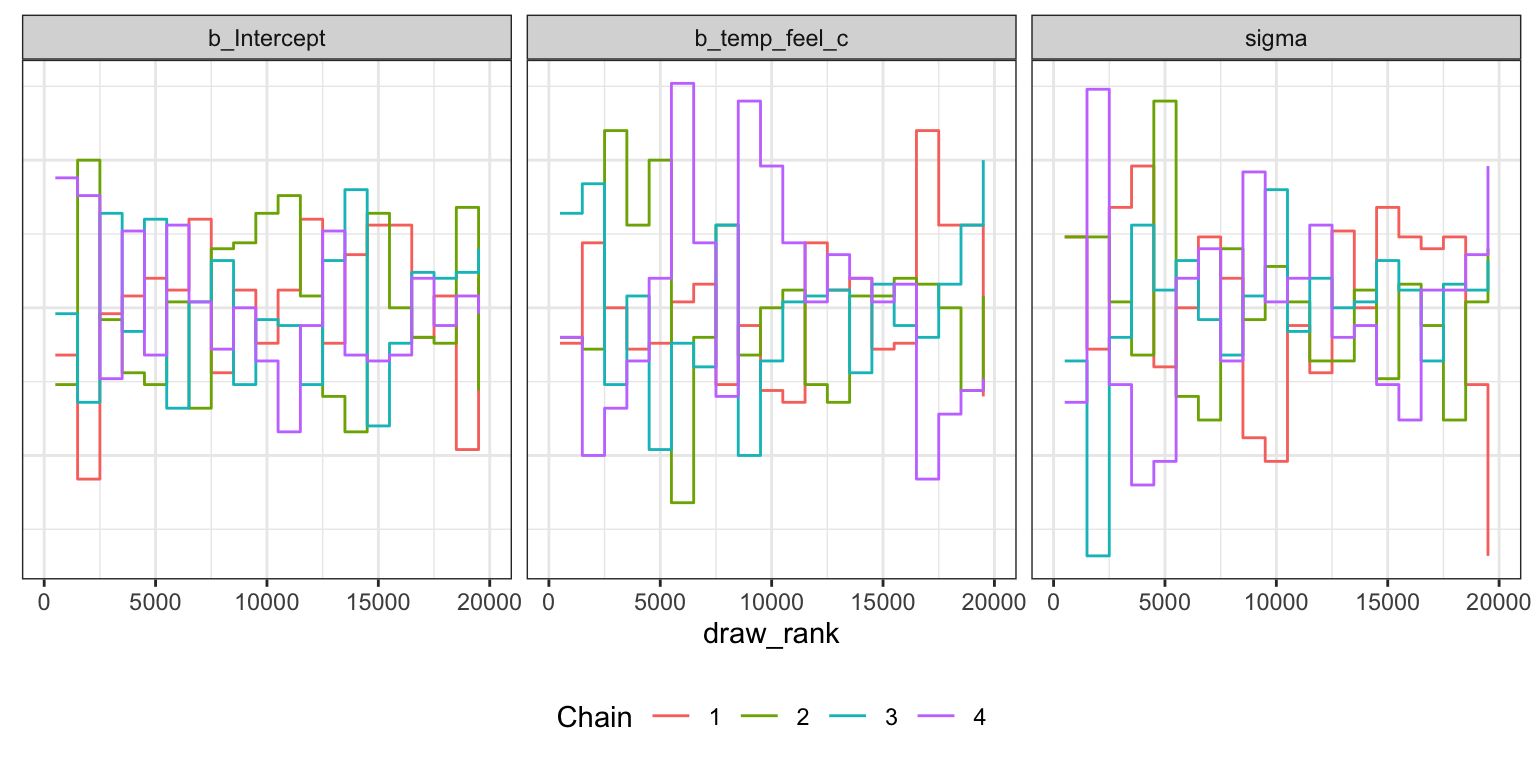##### Density plots bike_brms |> gather_draws(b_Intercept, b_temp_feel_c, sigma) |> ggplot(aes(x = .value, color = factor(.chain))) + geom_density() + labs(color = "Chain") + facet_wrap(vars(.variable), scales = "free") + theme(legend.position = "bottom")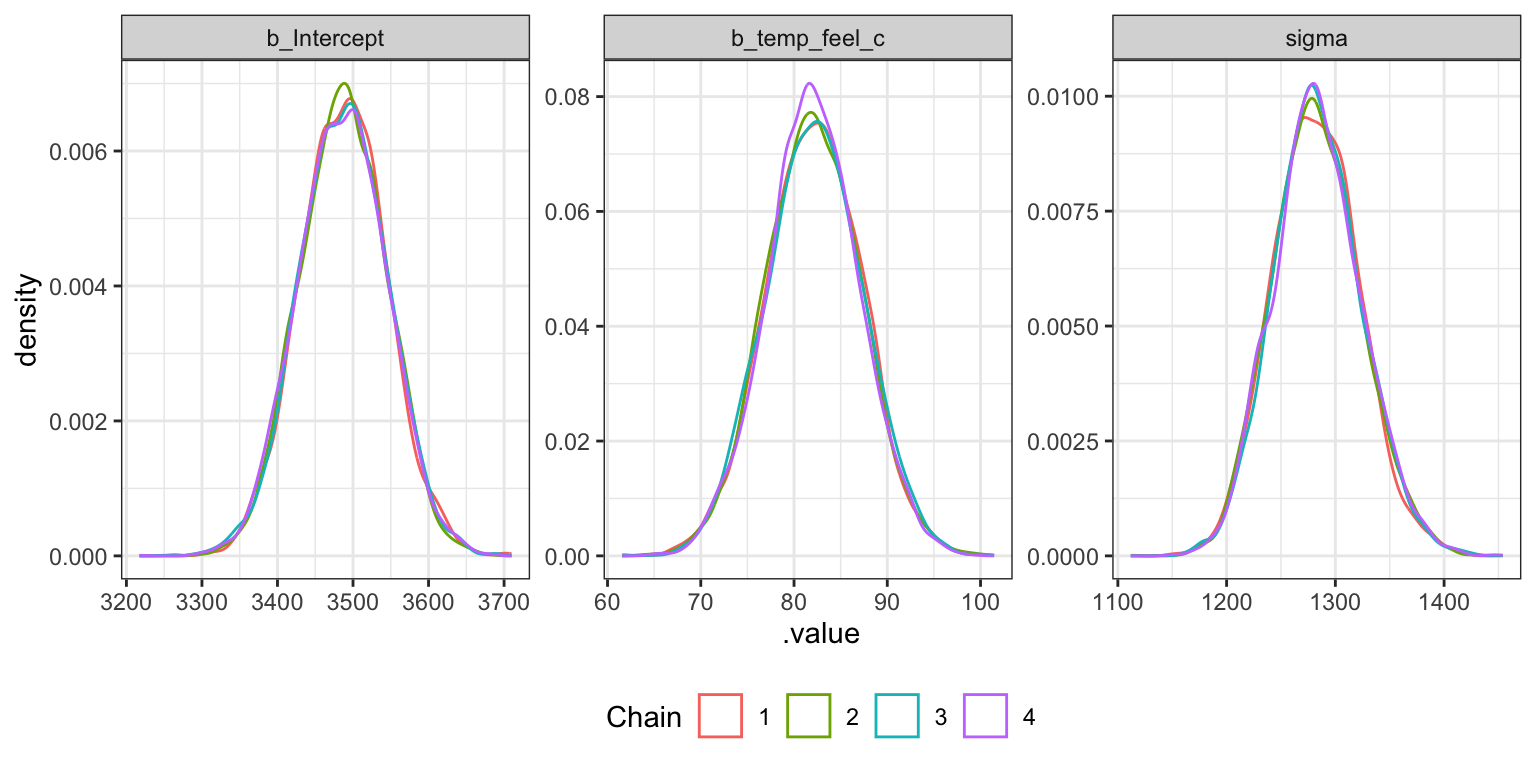##### Effective sample size and R-hat neff_ratio(bike_stan_samples, pars = c("beta0", "beta1", "sigma")) ## beta0 beta1 sigma ## 1.077305 1.012467 1.085847 rhat(bike_stan_samples, pars = c("beta0", "beta1", "sigma")) ## beta0 beta1 sigma ## 1.0001360 1.0000768 0.9999753 ##### Trace plots bike_stan_samples |> gather_draws(beta0, beta1, sigma) |> ggplot(aes(x = .iteration, y = .value, color = as.factor(.chain))) + geom_line(size = 0.05) + labs(color = "Chain") + facet_wrap(vars(.variable), scales = "free_y") + theme(legend.position = "bottom")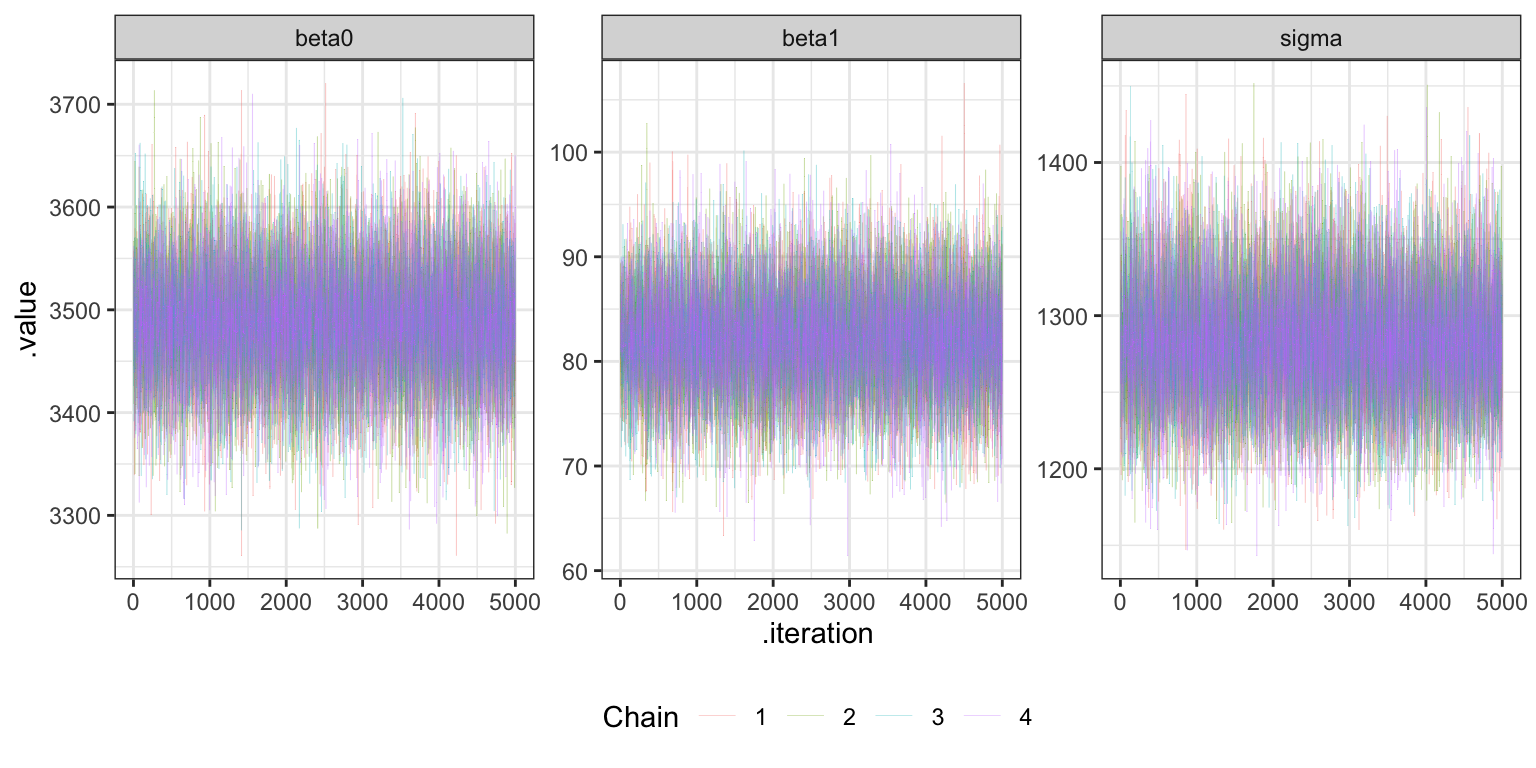##### Trank plots bike_stan_samples |> gather_draws(beta0, beta1, sigma) |> group_by(.variable) |> mutate(draw_rank = rank(.value)) |> ggplot(aes(x = draw_rank)) + stat_bin(aes(color = factor(.chain)), geom = "step", binwidth = 1000, position = position_identity(), boundary = 0) + labs(color = "Chain") + facet_wrap(vars(.variable)) + theme(legend.position = "bottom", axis.text.y = element_blank(), axis.title.y = element_blank(), axis.ticks.y = element_blank())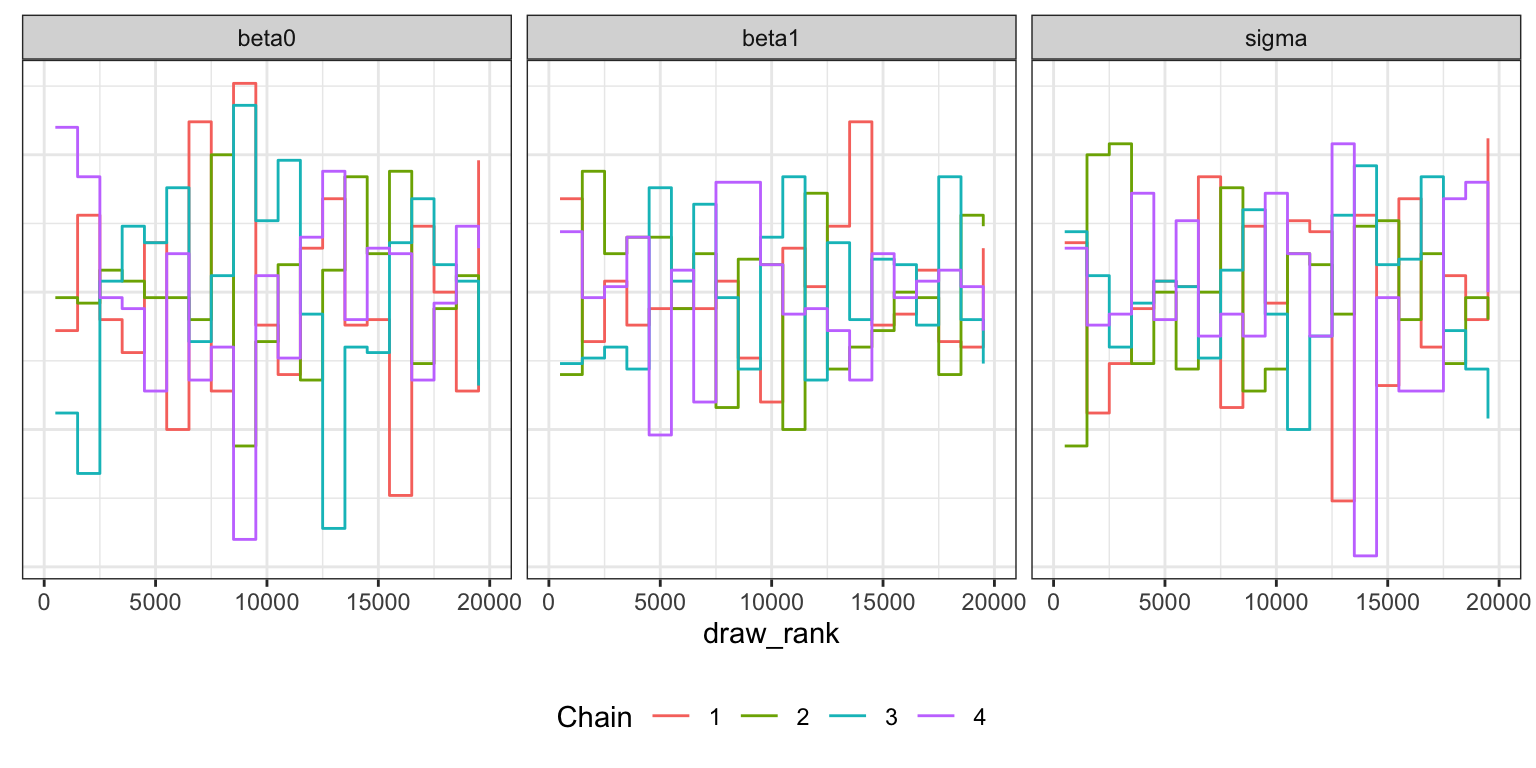##### Density plots bike_stan_samples |> gather_draws(beta0, beta1, sigma) |> ggplot(aes(x = .value, color = factor(.chain))) + geom_density() + labs(color = "Chain") + facet_wrap(vars(.variable), scales = "free") + theme(legend.position = "bottom")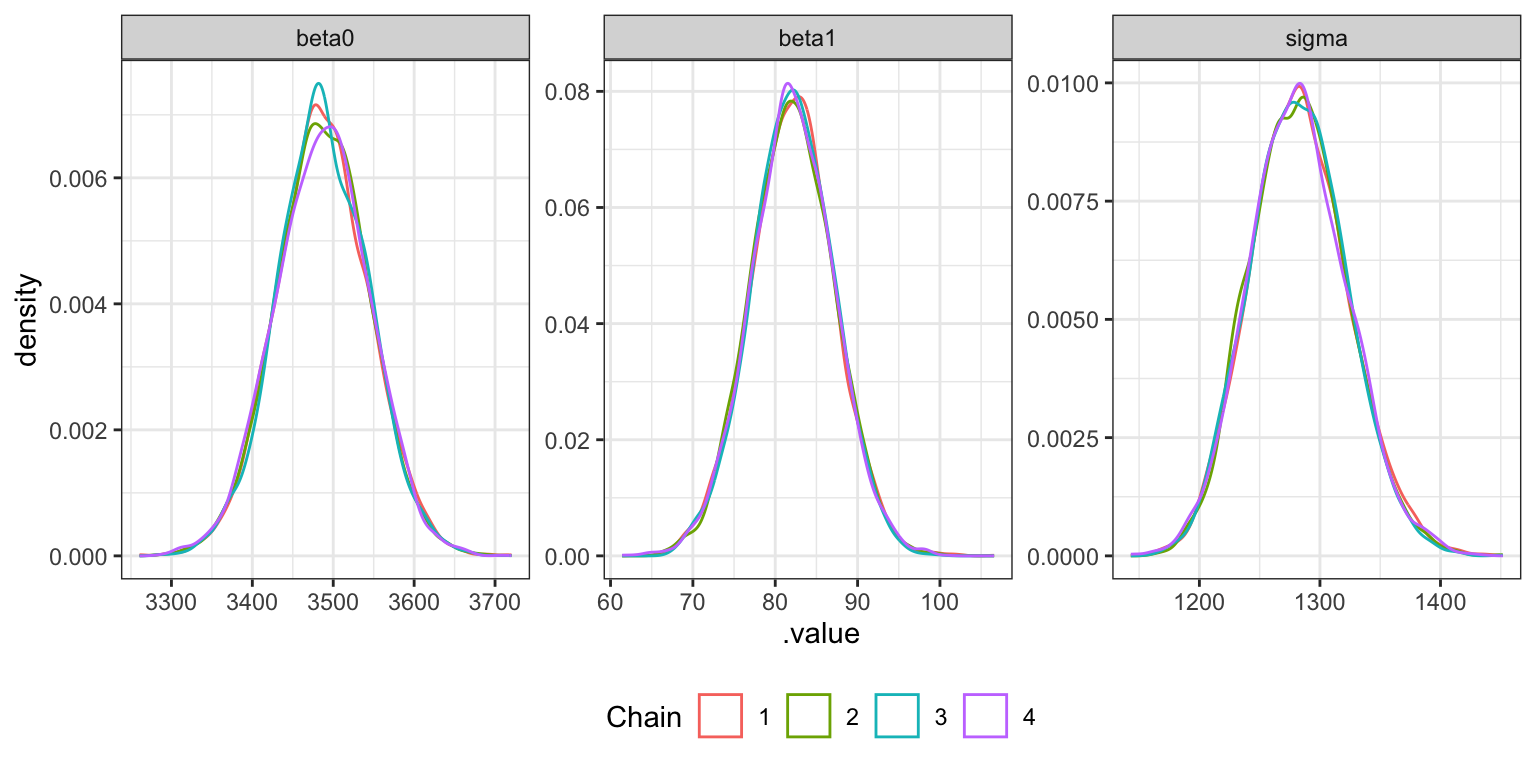## 9.4: Interpreting the posterior ### Parameter summaries tidy(bike_rstanarm, effects = c("fixed", "aux"), conf.int = TRUE, conf.level = 0.8) ## # A tibble: 4 × 5 ## term estimate std.error conf.low conf.high ## <chr> <dbl> <dbl> <dbl> <dbl> ## 1 (Intercept) 3487. 58.0 3413. 3561. ## 2 temp_feel_c 82.2 5.08 75.7 88.7 ## 3 sigma 1282. 40.9 1231. 1336. ## 4 mean_PPD 3487. 82.0 3382. 3593. bike_rstanarm |> gather_draws((Intercept), temp_feel_c, sigma) |> ungroup() |> mutate(.variable = fct_relevel(factor(.variable), c("(Intercept)", "temp_feel_c", "sigma"))) |> ggplot(aes(x = .value, fill = .variable)) + stat_halfeye(normalize = "xy") + scale_fill_manual(values = c(clrs, clrs, clrs), guide = "none") + facet_wrap(vars(.variable), scales = "free_x") + labs(x = "Parameter posterior") + theme(legend.position = "bottom") ## Warning: Unknown or uninitialised column: linewidth. ## Warning: Using the size aesthietic with geom_segment was deprecated in ggplot2 3.4.0. ## ℹ Please use the linewidth aesthetic instead. ## Warning: Unknown or uninitialised column: linewidth. ## Unknown or uninitialised column: linewidth.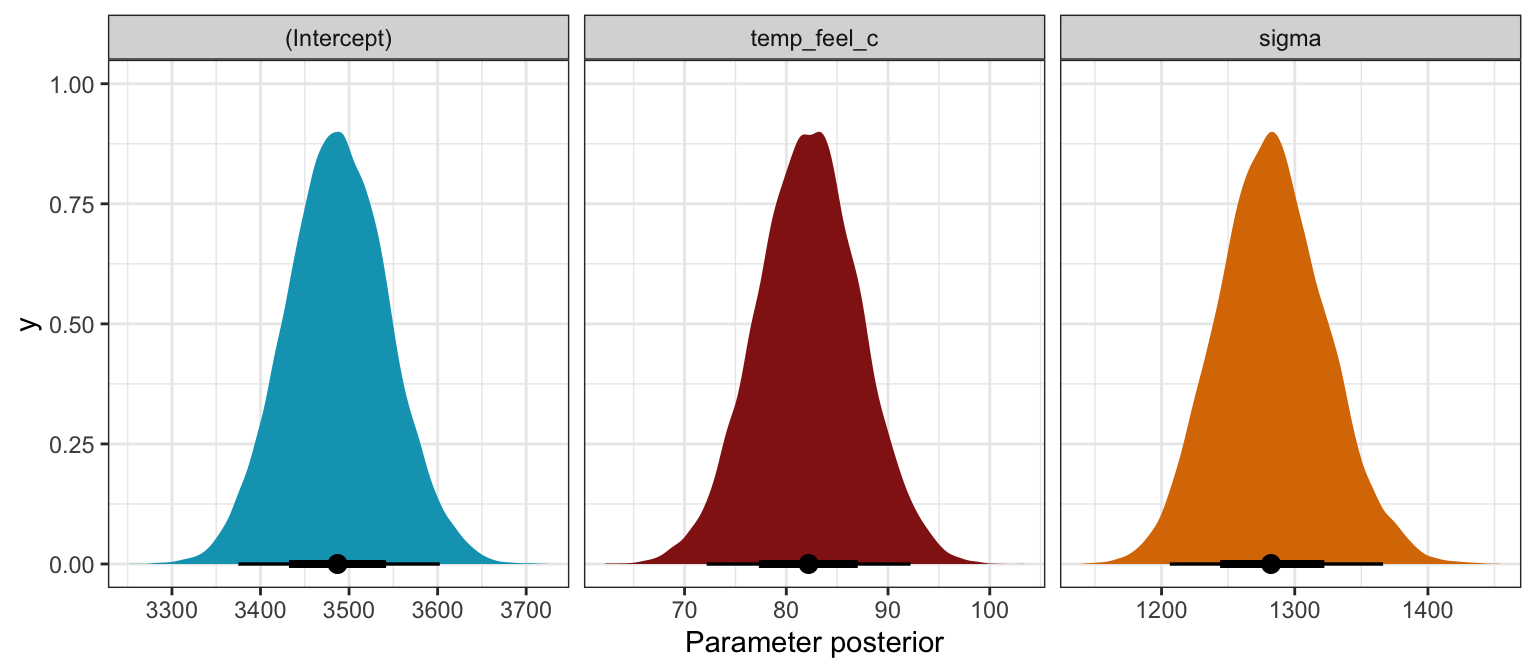tidy(bike_brms, conf.int = TRUE, conf.level = 0.8) |> select(-c(effect, component, group)) ## # A tibble: 3 × 5 ## term estimate std.error conf.low conf.high ## <chr> <dbl> <dbl> <dbl> <dbl> ## 1 (Intercept) 3487. 57.5 3414. 3561. ## 2 temp_feel_c 82.1 5.08 75.6 88.6 ## 3 sd__Observation 1283. 40.3 1232. 1336. bike_brms |> gather_draws(b_Intercept, b_temp_feel_c, sigma) |> ggplot(aes(x = .value, fill = .variable)) + stat_halfeye(normalize = "xy") + scale_fill_manual(values = c(clrs, clrs, clrs), guide = "none") + facet_wrap(vars(.variable), scales = "free_x") + labs(x = "Parameter posterior") + theme(legend.position = "bottom") ## Warning: Unknown or uninitialised column: linewidth. ## Unknown or uninitialised column: linewidth. ## Unknown or uninitialised column: linewidth.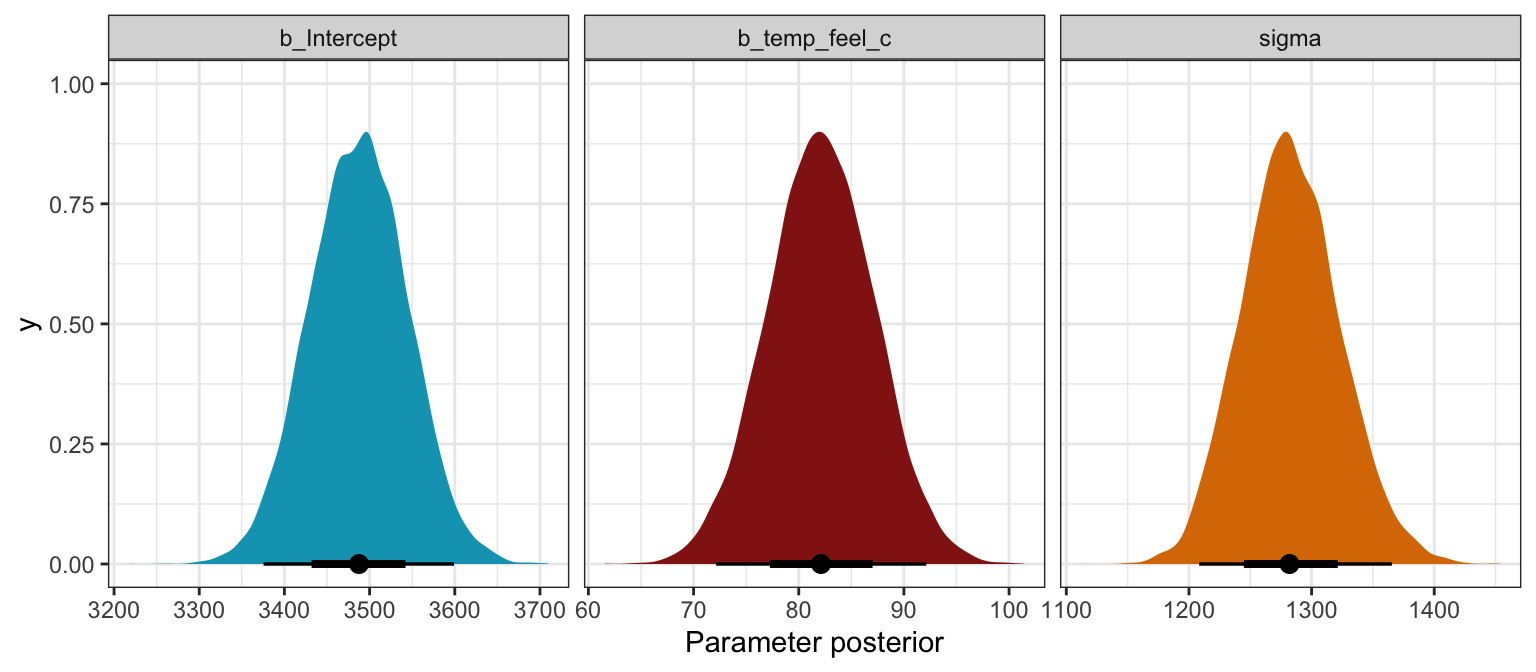bike_stan_samples$print(variables = c("beta0", "beta1", "sigma"),
"mean", "median", "sd", ~quantile(.x, probs = c(0.1, 0.9)))
##  variable    mean  median    sd     10%     90%
##     beta0 3487.16 3486.85 57.13 3414.32 3560.02
##     beta1   82.12   82.10  5.06   75.68   88.58
##     sigma 1282.72 1281.84 40.44 1231.39 1335.31
bike_stan_samples |>
gather_draws(beta0, beta1, sigma) |>
ggplot(aes(x = .value, fill = .variable)) +
stat_halfeye(normalize = "xy") +
scale_fill_manual(values = c(clrs, clrs, clrs), guide = "none") +
facet_wrap(vars(.variable), scales = "free_x") +
labs(x = "Parameter posterior") +
theme(legend.position = "bottom")
## Warning: Unknown or uninitialised column: linewidth.
## Unknown or uninitialised column: linewidth.
## Unknown or uninitialised column: linewidth.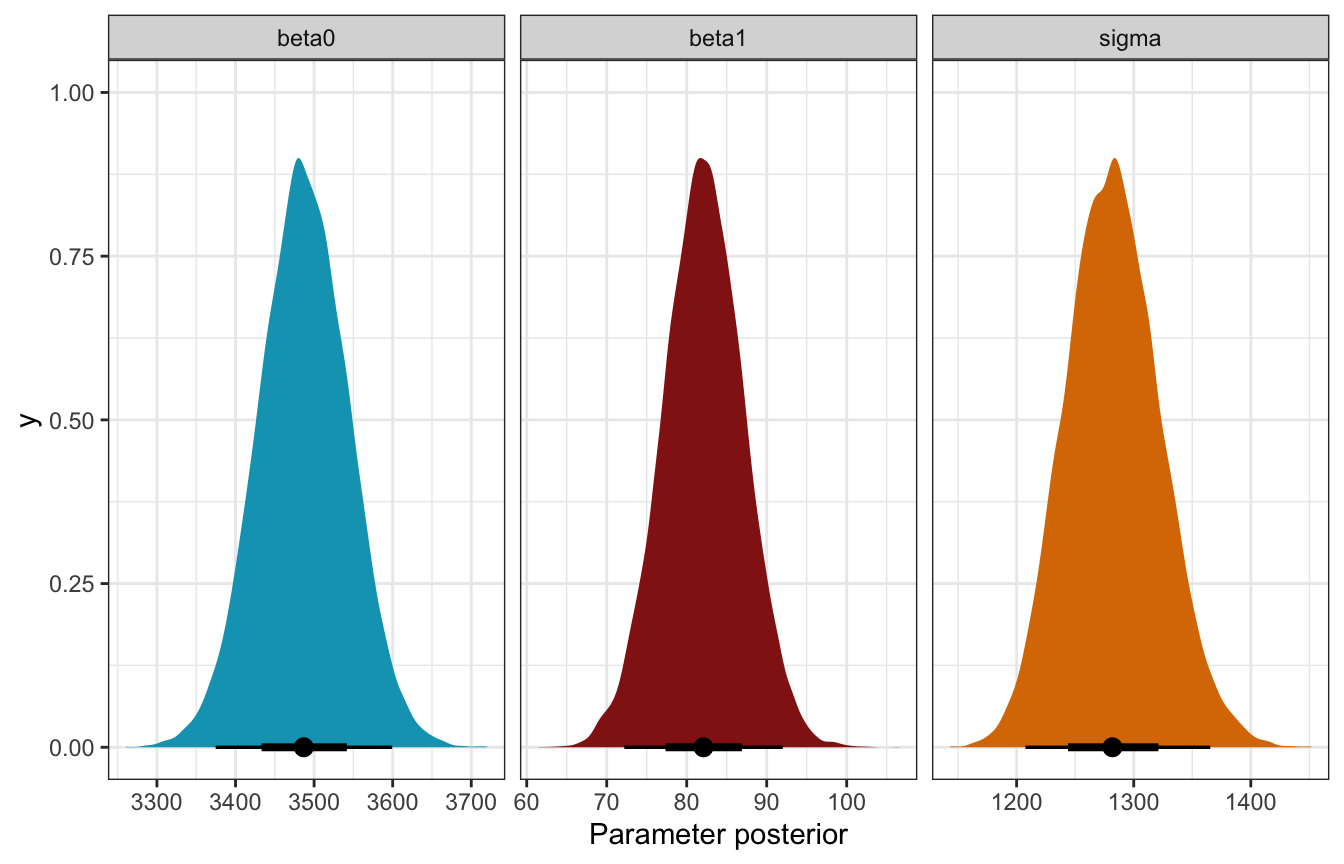### Fitted draws

bikes |>
ggplot(aes(x = temp_feel, y = rides)) +
geom_point(data = bikes, size = 0.5) +
geom_line(aes(y = .linpred, group = .draw), alpha = 0.2, size = 0.5, color = clrs) +
labs(x = "Temperature", y = "Rides")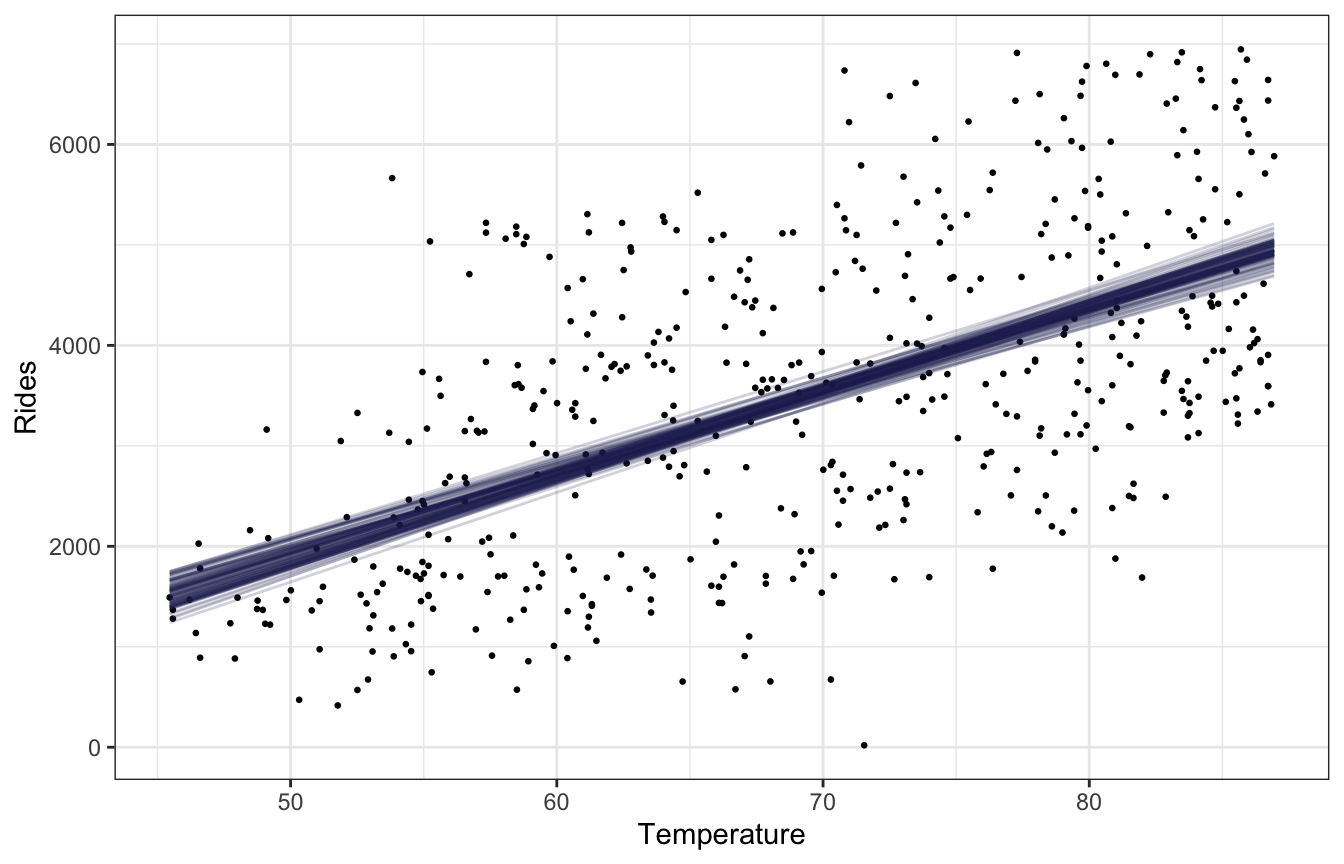bikes |>
ggplot(aes(x = temp_feel, y = rides)) +
geom_point(data = bikes, size = 0.5) +
geom_line(aes(y = .linpred, group = .draw), alpha = 0.2, size = 0.5, color = clrs) +
labs(x = "Temperature", y = "Rides")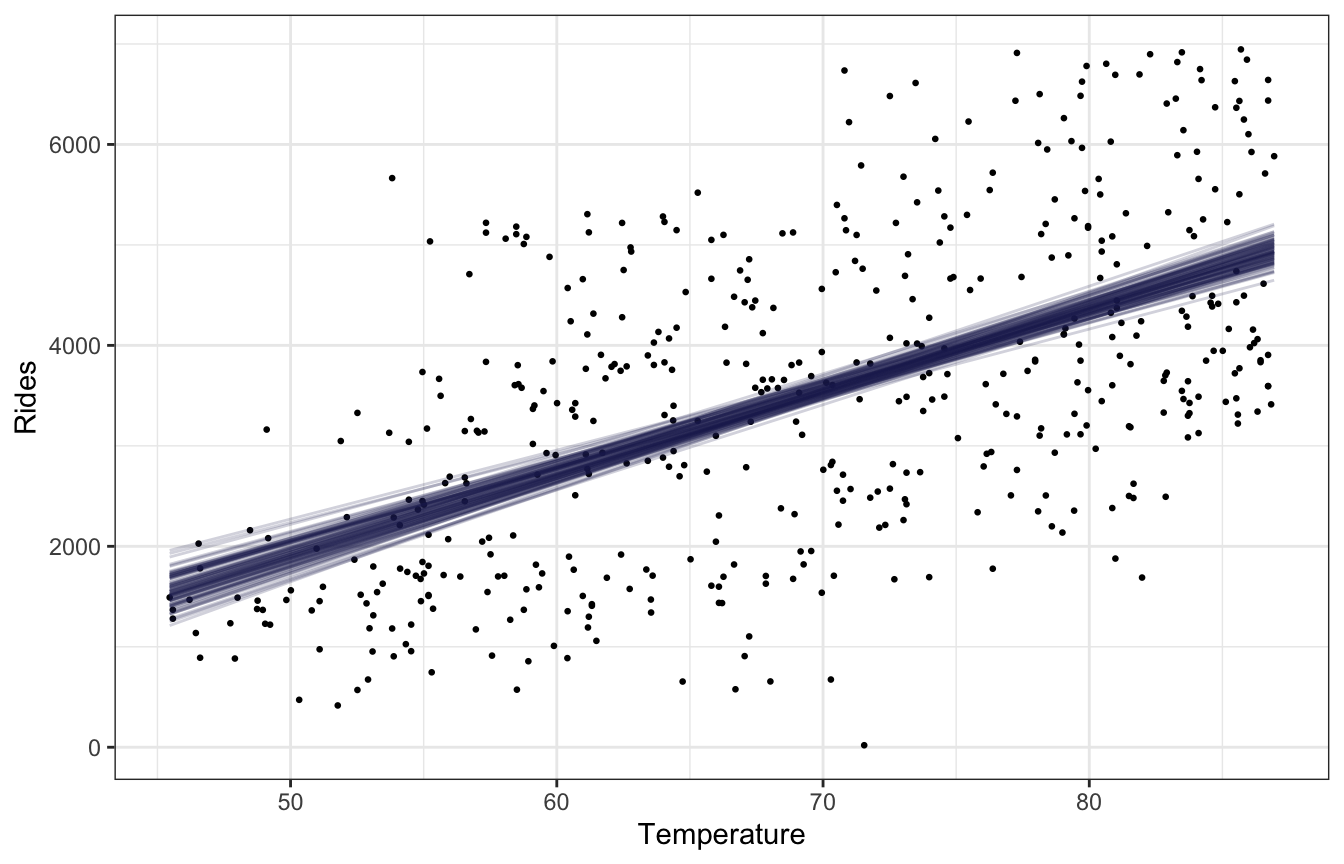bike_stan_samples |>
mean_qi() |>
bind_cols(bikes) |>
ggplot(aes(x = temp_feel, y = rides)) +
geom_point(size = 0.5) +
geom_line(aes(y = mu), color = clrs, size = 1) +
geom_ribbon(aes(ymin = .lower, ymax = .upper), fill = clrs, alpha = 0.2) +
labs(x = "Temperature", y = "Rides")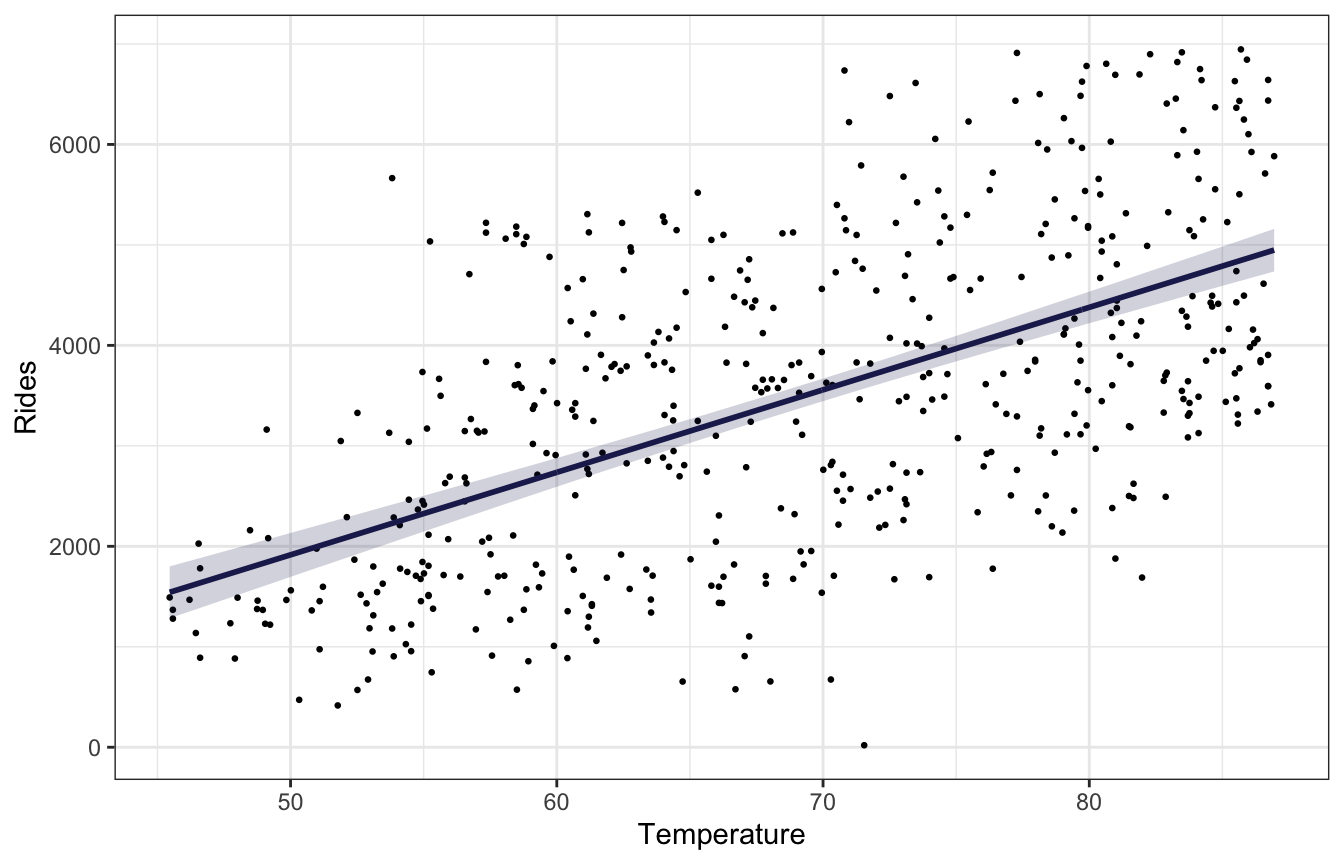There’s no easy way to replicate add_linpred_draws(..., ndraws = BLAH) with raw Stan like this without running the model again and generating a bunch of simulated mus based on some variable like n_sim that we build into the Stan model, but we can use the original 500 rows of data and use geom_lineribbon() instead of a spaghetti plot.

### Is β1 > 0?

Easily.

bike_rstanarm |>
count(temp_feel_c > 0) |>
mutate(prob = n / sum(n))
## # A tibble: 1 × 3
##   temp_feel_c > 0     n  prob
##   <lgl>             <int> <dbl>
## 1 TRUE              20000     1
bike_brms |>
count(b_temp_feel_c > 0) |>
mutate(prob = n / sum(n))
## # A tibble: 1 × 3
##   b_temp_feel_c > 0     n  prob
##   <lgl>               <int> <dbl>
## 1 TRUE                20000     1
bike_stan_samples |>
count(beta1 > 0) |>
mutate(prob = n / sum(n))
## # A tibble: 1 × 3
##   beta1 > 0     n  prob
##   <lgl>       <int> <dbl>
## 1 TRUE        20000     1

## 9.5: Posterior prediction

We could plug in some temperature, like 75, and get a predicted count of riders (values from the rstanarm model):

values_rstanarm <- bike_rstanarm |>
tidy() |>
split(~term)

b0 <- values_rstanarm$(Intercept)$estimate
b1 <- values_rstanarm$temp_feel_c$estimate
temp_mean <- temp_details$scaled_center b0 + (b1 * (75 - temp_mean)) ##  3968.317 But this ignores two types of uncertainty: • Sampling variability in the data, or in $$Y$$ • Posterior variability in parameters, or in $$\beta_0$$, $$\beta_1$$, and $$\sigma$$ p1 <- bike_rstanarm |> linpred_draws(newdata = tibble(temp_feel_c = (75 - temp_mean))) |> ggplot(aes(x = .linpred)) + stat_halfeye(fill = clrs) + labs(title = "Predicted riders at 75˚", subtitle = "µ only; posterior_linpred()", x = "Predicted riders", y = NULL) p2 <- bike_rstanarm |> predicted_draws(newdata = tibble(temp_feel_c = (75 - temp_mean))) |> ggplot(aes(x = .prediction)) + stat_halfeye(fill = clrs) + labs(title = "Predicted riders at 75˚", subtitle = "rnorm(µ, σ); posterior_predict()", x = "Predicted riders", y = NULL) p1 | p2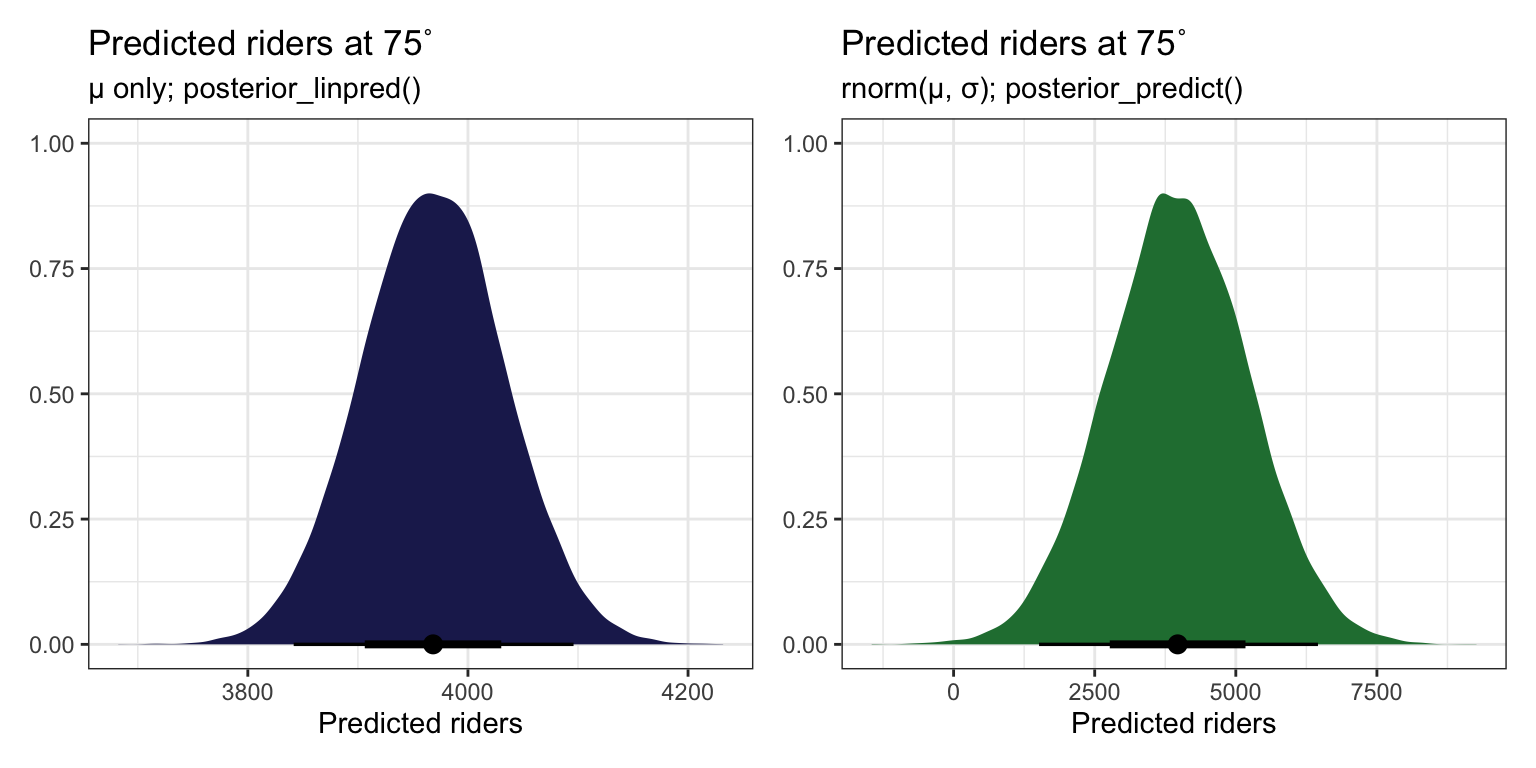p1 <- bike_brms |> linpred_draws(newdata = tibble(temp_feel_c = (75 - temp_mean))) |> ggplot(aes(x = .linpred)) + stat_halfeye(fill = clrs) + labs(title = "Predicted riders at 75˚", subtitle = "µ only; posterior_linpred()", x = "Predicted riders", y = NULL) p2 <- bike_brms |> predicted_draws(newdata = tibble(temp_feel_c = (75 - temp_mean))) |> ggplot(aes(x = .prediction)) + stat_halfeye(fill = clrs) + labs(title = "Predicted riders at 75˚", subtitle = "rnorm(µ, σ); posterior_predict()", x = "Predicted riders", y = NULL) p1 | p2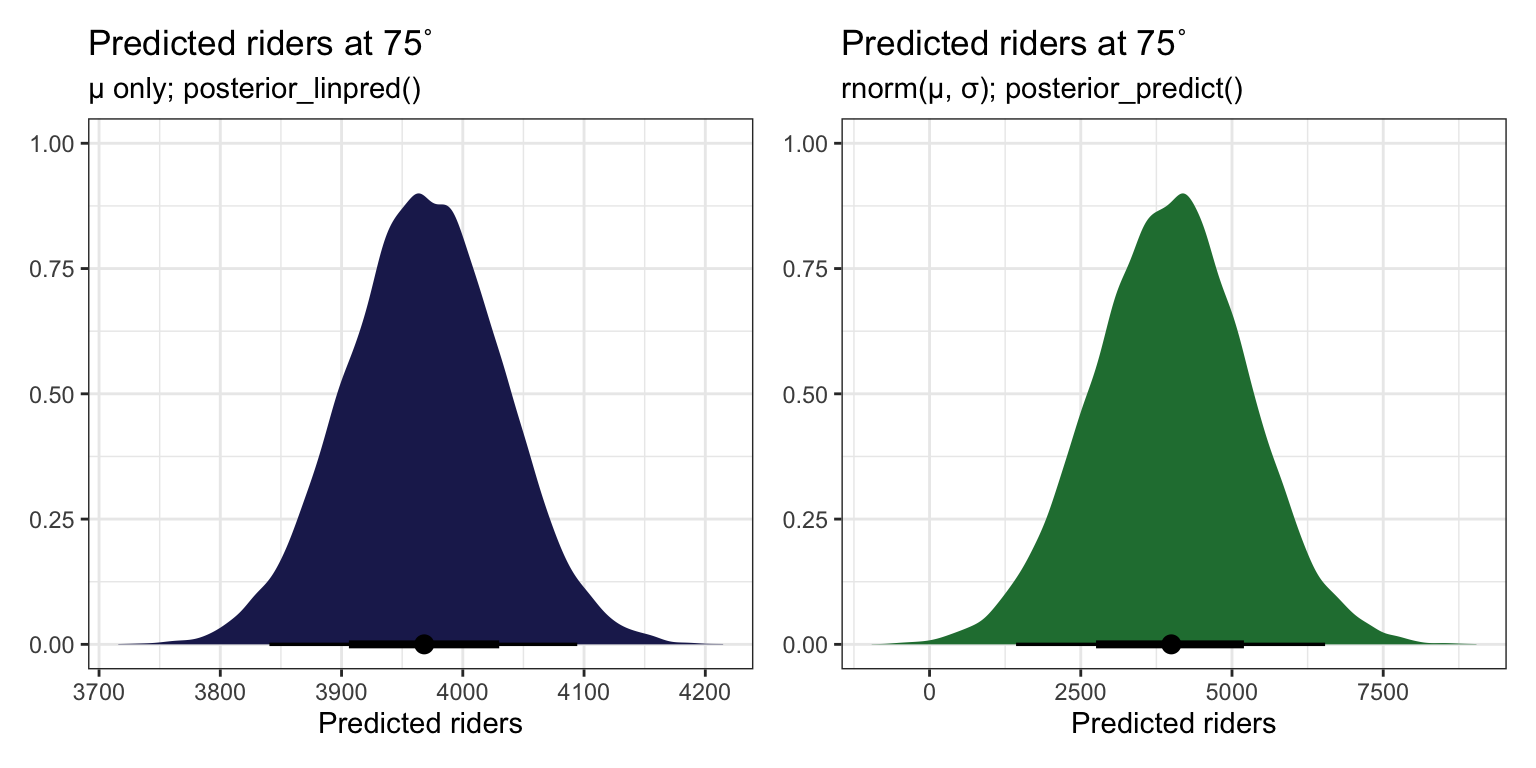AHH SO THIS IS HOW YOU DO newdata = WHATEVER WITH RAW STAN. Build mu (posterior_linpred()) on your own with the coefficients from each draw, then use that mu in rnorm() (posterior_predict()). You can then get the expectation of the posterior (posterior_epred()) by taking the average of that. predict_75 <- bike_stan_samples |> spread_draws(beta0, beta1, sigma) |> mutate(mu = beta0 + (beta1 * (75 - temp_mean)), # like posterior_linpred() y_new = rnorm(n(), mean = mu, sd = sigma)) # like posterior_predict() p1 <- predict_75 |> ggplot(aes(x = mu)) + stat_halfeye(fill = clrs) + labs(title = "Predicted riders at 75˚", subtitle = "µ only; like posterior_linpred()", x = "Predicted riders", y = NULL) p2 <- predict_75 |> ggplot(aes(x = y_new)) + stat_halfeye(fill = clrs) + labs(title = "Predicted riders at 75˚", subtitle = "rnorm(µ, σ); like posterior_predict()", x = "Predicted riders", y = NULL) p1 | p2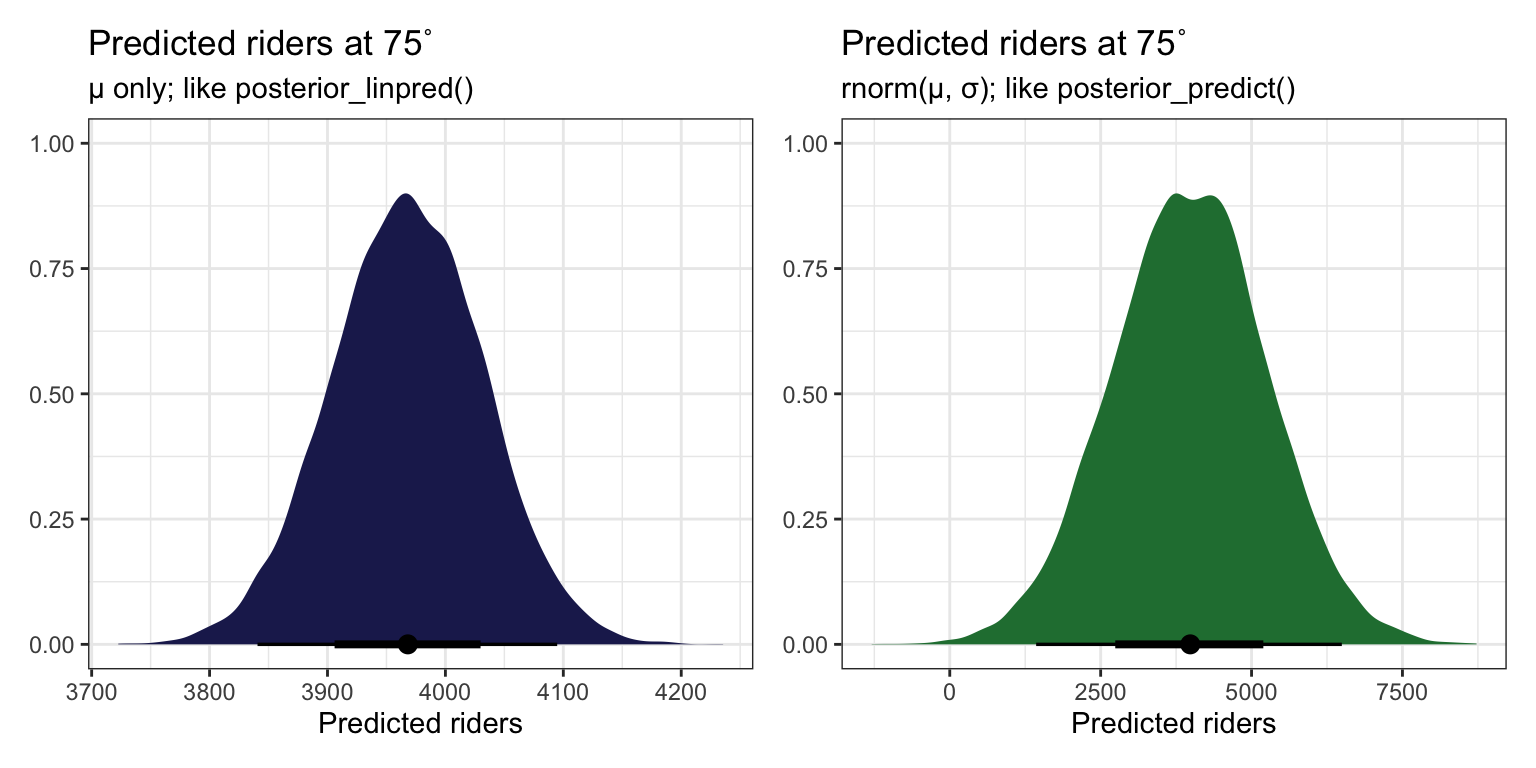predict_75 |> summarize(epred = mean(y_new)) ## # A tibble: 1 × 1 ## epred ## <dbl> ## 1 3974. ## 9.6: Sequential regression modeling Bayesianism is all about updating. What does this relationship between temperature and ridership as data is slowly collected, like after 30 days, 60 days, and 500 days? How does the posterior evolve and settle? I’ll do this just with brms: priors <- c(prior(normal(5000, 1000), class = Intercept), prior(normal(100, 40), class = b, coef = "temp_feel_c"), prior(exponential(0.0008), class = sigma)) bike_phases <- tribble( ~phase, ~data, 1, slice(bikes, 1:30), 2, slice(bikes, 1:60), 3, bikes ) |> mutate(model = map(data, ~{ brm( bf(rides ~ temp_feel_c), data = ., family = gaussian(), prior = priors, chains = 4, iter = 5000*2, seed = BAYES_SEED, backend = "cmdstanr", refresh = 0 )}) ) ## Start sampling ## Start sampling ## Start sampling bike_phase_draws <- bike_phases |> mutate(draws = map(model, ~spread_draws(., b_temp_feel_c))) phases_coefs <- bike_phase_draws |> mutate(coef_plot = map2(draws, phase, ~{ ggplot(.x, aes(x = b_temp_feel_c)) + stat_halfeye(fill = clrs) + coord_cartesian(xlim = c(-10, 100)) + labs(title = paste("Phase", .y)) })) wrap_plots(phases_coefs$coef_plot)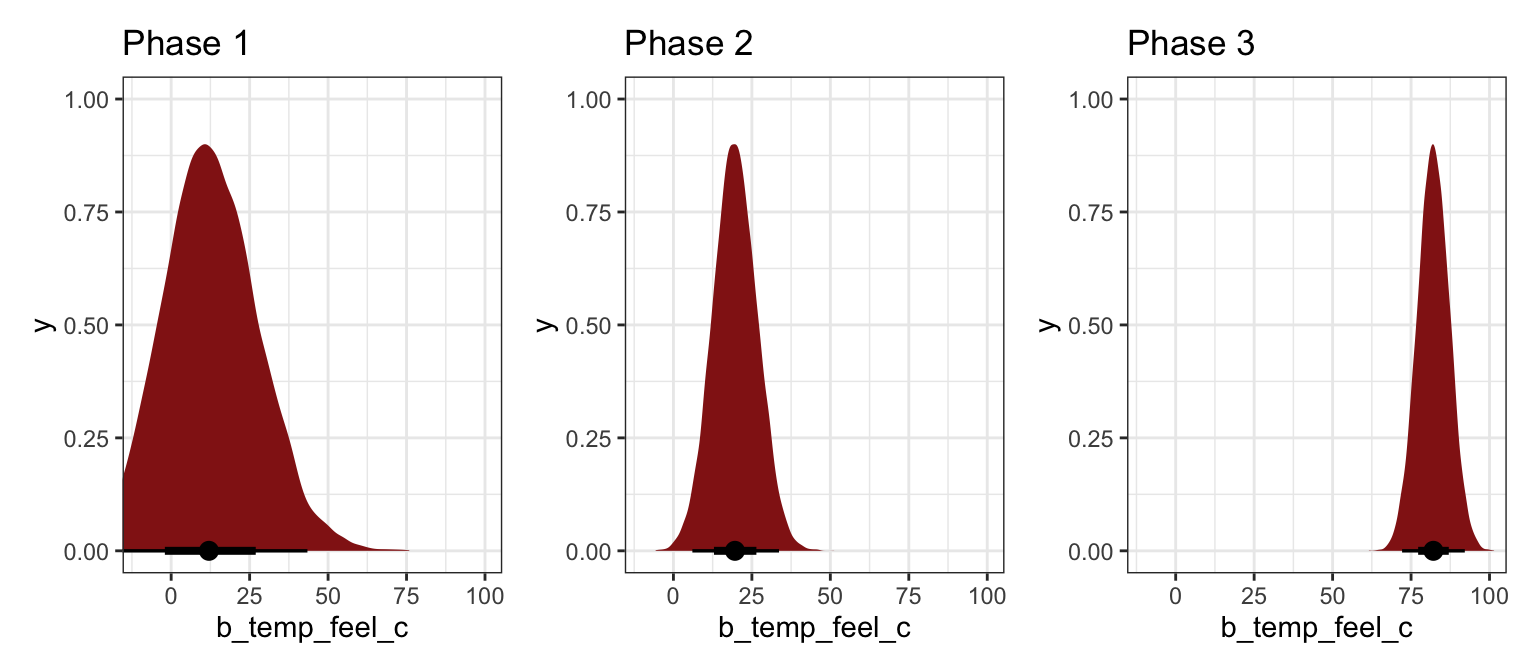bike_phase_preds <- bike_phases |>
mutate(preds = map2(data, model, ~{
.x |>
ungroup()
}))

phases_preds <- bike_phase_preds |>
mutate(pred_plot = pmap(list(data, preds, phase), ~{
ggplot(..2, aes(x = temp_feel, y = rides)) +
geom_point(data = ..1, size = 0.5) +
geom_line(aes(y = .linpred, group = .draw),
alpha = 0.2, size = 0.5, color = clrs) +
labs(title = paste("Phase", ..3)) +
coord_cartesian(xlim = c(40, 90), ylim = c(0, 7000))
}))

wrap_plots(phases_preds\$pred_plot)